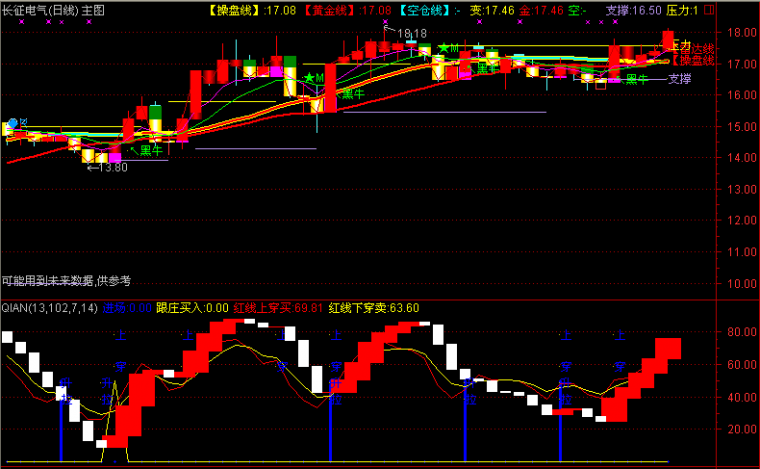﻿ 宝华提醒指标公式 源码-通达信公式 －程序化交易（CXH99.COM）

# 宝华提醒指标公式 源码[通达信公式]

{宝华提醒}
VAR1:=((LLV(LOW,5) = LLV(LOW,45)) AND (CLOSE >= (OPEN * 1.04)));
VAR2:=(CLOSE > ((REF(HIGH,1) + REF(LOW,1)) / 2));
VAR3:=REF(CLOSE,1);
VAR4:=((SMA(MAX((CLOSE - VAR3),0),6,1) / SMA(ABS((CLOSE - VAR3)),6,1)) * 100);{宝华提醒}
VAR1:=((LLV(LOW,5) = LLV(LOW,45)) AND (CLOSE >= (OPEN * 1.04)));
VAR2:=(CLOSE > ((REF(HIGH,1) + REF(LOW,1)) / 2));
VAR3:=REF(CLOSE,1);
VAR4:=((SMA(MAX((CLOSE - VAR3),0),6,1) / SMA(ABS((CLOSE - VAR3)),6,1)) * 100);

(注：由于人数限制，QQ或微信请选择方便的一个联系我们就行，加好友时请简单备注下您的需求，否则无法通过。谢谢您！)

【字体： 】【打印文章】【查看评论

没有相关内容